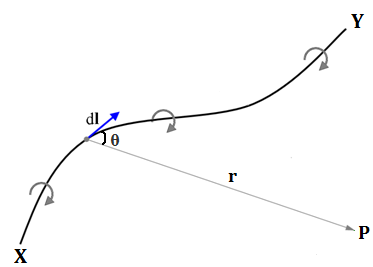# Biot and Savart law

Biot and Savart law gives the quantitative measurement of magnetic field due to a current carrying conductor.

Let us consider a conductor XY flowing current I through it. Since, the current is flowing through the condutor, magnetic field is produced at point P. Let dl be the small element of which make angle  with the line joining P as shown in figure. The distance from dl to P is supposed to be r.Now, according to Biot and Savart law, the magnetic field due to a current carrying element is:

1. Directly proportional to the magnitude of current passed. i.e., dB∝I……(i)
2. Directly proportional to the length of the element. i.e., dB∝dl……(ii)
3. Directly proportional to the sine of angle between the conductor and line joining r. i.e., dB∝sinθ……(iii)
4. Inversely proportional to square of the line between the centre of the element and the point P. i.e., dB∝ $\frac{1}{ \text{r}^2}……(iv)$

combining these relations, we get,
$$\text{dB} \propto \frac{\text{Idlsin} \theta }{ \text{r}^2}$$
$$\text{or, dB} = \text{k}\frac{\text{Idlsin} \theta }{ \text{r}^2}$$
Here, ‘k’ is a proportionality constant.
In S.I unit for air, $\text{k} = \frac{ \mu_o}{4 \pi }$
Where, μ= 4π×10-7 wbm-1A-1(TmA-7) called permeability of air.

$$\therefore \text{dB} = \frac{ \mu_o}{4 \pi } \times \frac{\text{Idlsin} \theta }{ \text{r}^2}$$

Vectorically,

$$\vec{\text{dB}} = \frac{ \mu_o}{4 \pi } \times \frac{\text{I} \vec{dl} \times \vec {r}}{r^3}$$

In C.G.S system for air, k=1
$$\therefore\text{dB} = \text{k}\frac{\text{Idlsin} \theta }{ \text{r}^2}$$

The direction of dB can be determined by using right hand thumb rule.

Do you like this article ? If yes then like otherwise dislike : 0

#### One Response to “Biot and Savart law”

1.Prawin Pokhrel

good!!!